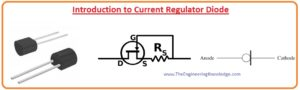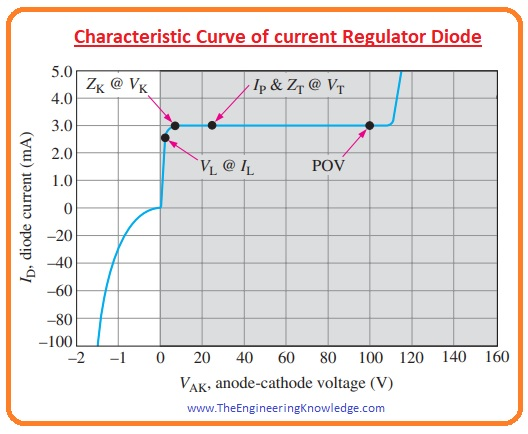Hello friends, I hope you all are doing great. In today’s tutorial, we will have a look at Introduction to Current Regulator Diode. The current regulating diode is also known as a current limiting diode or constant current diode that used to limit the current for specific devices irrespective of variation in voltage. These diodes comprise of the N channel Junction Field Effect Transistor having a gate terminal connected with the source. This construction makes it similar to the two terminals’ current sources or like the Zener diode.

As we know that Zener diode regulates the voltage this diode regulates the current similar to Zener diode. Irrespective variation in input voltage this diode maintains the current values constant. In today’s post, we will have a detailed look at its working, structure, applications and some other related parameters. So let’s get started with Introduction to Current Regulator Diode.

#### Introduction to Current Regulator Diode

• The current regulator diode is used to regulate the current as the Zener diode regulates the voltage.
• The symbol of the current regulator diode is shown in the below figure.
• These diodes are operated in forward biasing condition use voltage of 1.5 volts to volts.
• The current generated for forward biasing is known as forward current and denoted as  Ip. We discuss it in detail.
• These diodes, when used in electronic circuits for operation of LED, optocoupler and battery charges, provides stability, protection, and regulation.
• Normally one volt to hundred volts input voltage diode can regulate the current.
• With the variations in voltages at input, load resistance variations, this diode maintains the current value.
• This diode is usually exiting in TO-206AA and in MIL-S-19500.#### Characteristic Curve of Current Regulator Diode

• In the below figure characteristic curve of current regulator, a diode is shown. This curve is divided into different regions.
• In a shaded part of the curve diode is operating in forward biasing condition, the value of forward current is constant for voltage range of 1.5 volts to six volts.
• The forward current is known as regulator current and denoted as Ip.
• For instance, for diode series 1N5283 to 1N5314 the value of regulator current is from 220 milliamperes to 4.7 milliamperes.
• To get a large value of current these diodes are used in parallel combination.
• For this diode there is no specific reverse breakdown, this the reverse current start to upsurge for the VAK of less than zero volts.
• Like other diodes, this diode does not operate in reverse biased conditions.
• In forward biased conditions the regulation process of diode start at limiting voltage VL, to the POV or peak operating voltage.
• You can observe from the curve that between points Vk and POV the current is constant.
• VT is the test voltage for this voltage IP and ZT mentioned on the datasheet of a diode.
• The value of impedance ZT is 235 KΩ to 25 MΩ for diode series 1N5283 to 1N5314.#### Current Regulator Diode Features

• These are some features of the current Regulator Diode.
• This diode has two pinouts and exits in a closed casing.
• Its tolerance is puls-minus ten percent.
• It can operate for a voltage range of one volt to a hundred volts.
• It shows stable behavior for variation in temperature values.
• Its peak functioning voltage is one hundred volts.
• Its reverse current value is one hundred volts.
• Its thermal resistance value is one hundred C/w.
• Its storage temperature rating is -55 to 200 centigrade.
• Its power dissipation is thirty milliwatts.

• These are some benefits of the Current Regulator Diode.
• Its circuit is very simple exits in series combinations and no need of any additional voltage source.
• It continues operation for low voltage, battery circuitry, and provides protection for large voltage harmonics protection.
• With the increment of temperature, its circuitry maintains operations.

#### Current Regulator Diode Applications

• These are some applications of the Current Regulator Diode.
• It operates in circuits where a large length of the LED string is used and in voltage variable sources such as bulbs.
• To provide input to the solid-state relays these diodes are used.